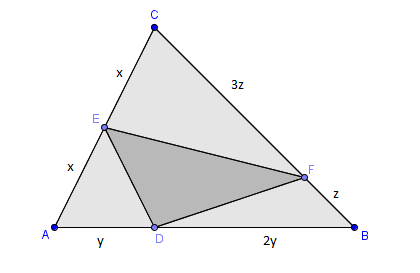# One, two, three, let's do itIn the diagram, relative lengths of some line segments are as follows:

$\begin{array}{c}&CE=AE, &DB=2AD, &CF=3BF. \end{array}$

If the area of $\triangle ABC$ is $24,$ what is the area of $\triangle DEF?$

×